# Question: Determine the set of rationalizable strategies for each of thefollowing games.Player 2Player 2(0, 4)(3, 3)(4, 0)Player 2(2,0)(3, 4)(1,3)(4, 2)(2, 3)(4,0)(3, 3)(0, 4)(1,1)Player 1Player 1(1,2)(0,2) | (3,0)Player 2DD(5, 1) | (0, 2) | Player 1(4, 6)(3, 5)(6, 3)(0, 1)(2,1)Player 2(8, 6)(1,0)(0,8)(8, 2)(0, 1)(2, 6)(5, 1)(4, 4)(1,0)Player 1(6,0)(2,8)DDPlayer 2(8, 10)(6, 4)(2, 2)(0,0)(0,0)(3,3)(4, 1)(8, 5)Player 1Player 1BBPlayer 2Player 2(8, 10)(6, 4)(3, 10)(6, 4)(4, 1)(8, 5)(4, 1)(8, 5)Player 1APlayer 1BB

Question
10 views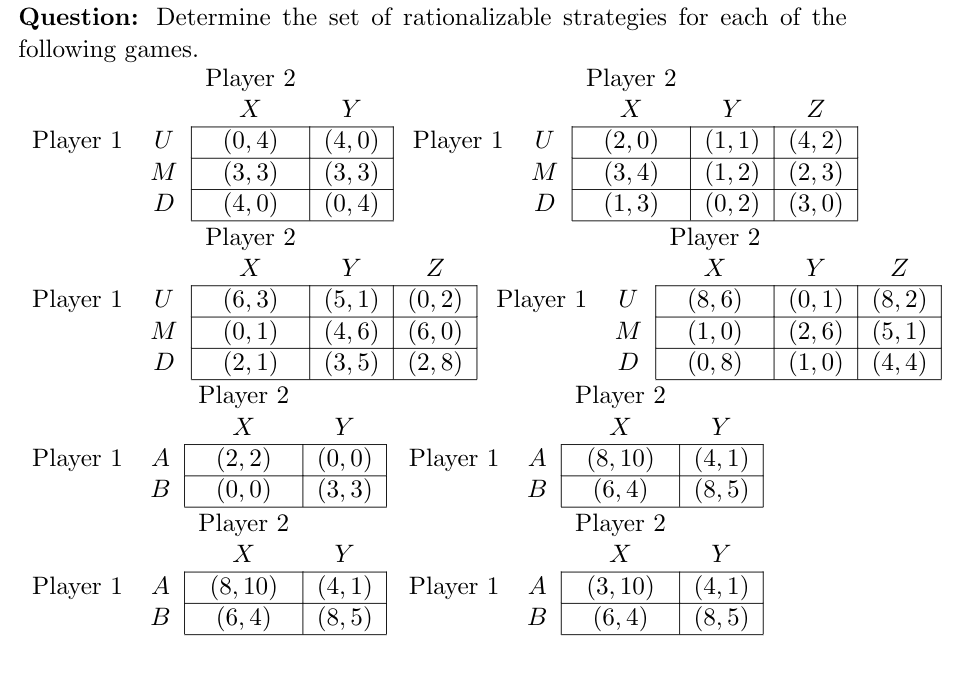help_outlineImage TranscriptioncloseQuestion: Determine the set of rationalizable strategies for each of the following games. Player 2 Player 2 (0, 4) (3, 3) (4, 0) Player 2 (2,0) (3, 4) (1,3) (4, 2) (2, 3) (4,0) (3, 3) (0, 4) (1,1) Player 1 Player 1 (1,2) (0,2) | (3,0) Player 2 D D (5, 1) | (0, 2) | Player 1 (4, 6) (3, 5) (6, 3) (0, 1) (2,1) Player 2 (8, 6) (1,0) (0,8) (8, 2) (0, 1) (2, 6) (5, 1) (4, 4) (1,0) Player 1 (6,0) (2,8) D D Player 2 (8, 10) (6, 4) (2, 2) (0,0) (0,0) (3,3) (4, 1) (8, 5) Player 1 Player 1 B B Player 2 Player 2 (8, 10) (6, 4) (3, 10) (6, 4) (4, 1) (8, 5) (4, 1) (8, 5) Player 1 A Player 1 B B fullscreen
check_circle

star
star
star
star
star
1 Rating
Step 1

Hey, Thank you for the question. According to our policy we can only answer 3 subparts per question. So, we are solving the 1st three for you. If you need further help, post the question again and mention the subpart you want us to solve.

There are two players in all the strategies: Player 1 and player 2.

The payoffs are written in this format – (player 1, player 2). We see player 1’s payoff row wise and player 2’s payoffs column wise.

We will assume that player 1 chooses the strategy 1st. So, it will for sure want to maximize its payoffs (row-wise). Similarly, player 2 will also maximize its payoffs (column-wise).

Step 2

1)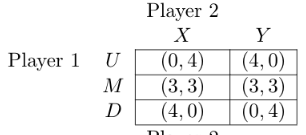In this case we can see none of the payoffs are maximized or are equal. If we see for player 1(row-wise 1st element): strategy U – the payoffs are 0 and 4, Strategy M – 3 and 3, Strategy D – 4 and 0. None of the payoffs are maximized in terms of others.  Example- for strategy U and M – 0>3 but 4 is not >3. For U and D – 0 is not >3 but 4>0. Similarly for M and D – 3 is not > 4 but 3>0. So, it can be said none of the strategy is dominated.

Similarly, see for player 2(Column-wise 2nd element): For strategy X and Y – 4>0, 3=3 but 0 is not greater than 4. So, it can be said none of the strategy is dominated.

Therefore, rationalizable strategy for this game is the same. That is:Step 3

2)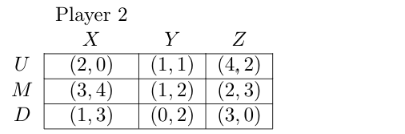Following the same method: for player 1 : Strategy U and D- 2>1, 1>0 , 4> 3. Therefore, D is dominated by U and we can eliminate it.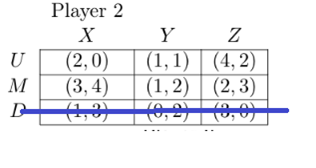Now the game left is: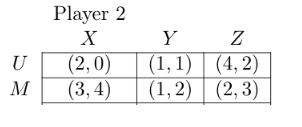From player 1’s side neither U nor M dominates. So, looking from player 2’s side (column-wise and 2nd element) – Strategy Y and Z – 2>1, 3>2 . Therefore, Z dominates Y and we can eliminate Z. From X and Y none of them dominates. So, rationalizable strategy for this game is: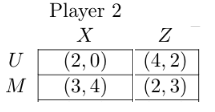...

### Want to see the full answer?

See Solution

#### Want to see this answer and more?

Solutions are written by subject experts who are available 24/7. Questions are typically answered within 1 hour.*

See Solution
*Response times may vary by subject and question.
Tagged in